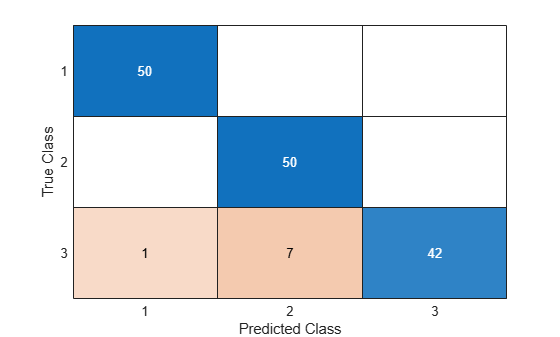# predict

Label new data using semi-supervised self-trained classifier

Since R2020b

## Syntax

``label = predict(Mdl,X)``
``[label,score] = predict(Mdl,X)``

## Description

example

````label = predict(Mdl,X)` returns a vector of predicted class labels for the data in the table or matrix `X`, based on the semi-supervised self-trained classifier `Mdl`.```
````[label,score] = predict(Mdl,X)` also returns a matrix of scores indicating the likelihood that a label comes from a particular class. For each observation in `X`, the predicted class label corresponds to the maximum score among all classes.```

## Examples

collapse all

Use both labeled and unlabeled data to train a `SemiSupervisedSelfTrainingModel` object. Label new data using the trained model.

Randomly generate 15 observations of labeled data, with 5 observations in each of three classes.

```rng('default') % For reproducibility labeledX = [randn(5,2)*0.25 + ones(5,2); randn(5,2)*0.25 - ones(5,2); randn(5,2)*0.5]; Y = [ones(5,1); ones(5,1)*2; ones(5,1)*3];```

Randomly generate 300 additional observations of unlabeled data, with 100 observations per class.

```unlabeledX = [randn(100,2)*0.25 + ones(100,2); randn(100,2)*0.25 - ones(100,2); randn(100,2)*0.5];```

Fit labels to the unlabeled data by using a semi-supervised self-training method. The function `fitsemiself` returns a `SemiSupervisedSelfTrainingModel` object whose `FittedLabels` property contains the fitted labels for the unlabeled data and whose `LabelScores` property contains the associated label scores.

`Mdl = fitsemiself(labeledX,Y,unlabeledX)`
```Mdl = SemiSupervisedSelfTrainingModel with properties: FittedLabels: [300x1 double] LabelScores: [300x3 double] ClassNames: [1 2 3] ResponseName: 'Y' CategoricalPredictors: [] Learner: [1x1 classreg.learning.classif.CompactClassificationECOC] ```

Randomly generate 150 observations of new data, with 50 observations per class. For the purposes of validation, keep track of the true labels for the new data.

```newX = [randn(50,2)*0.25 + ones(50,2); randn(50,2)*0.25 - ones(50,2); randn(50,2)*0.5]; trueLabels = [ones(50,1); ones(50,1)*2; ones(50,1)*3];```

Predict the labels for the new data by using the `predict` function of the `SemiSupervisedSelfTrainingModel` object. Compare the true labels to the predicted labels by using a confusion matrix.

```predictedLabels = predict(Mdl,newX); confusionchart(trueLabels,predictedLabels)```Only 8 of the 150 observations in `newX` are mislabeled.

## Input Arguments

collapse all

Semi-supervised self-training classifier, specified as a `SemiSupervisedSelfTrainingModel` object returned by `fitsemiself`.

Predictor data to be classified, specified as a numeric matrix or table. Each row of `X` corresponds to one observation, and each column corresponds to one variable.

If you trained `Mdl` using matrix data (`X` and `UnlabeledX` in the call to `fitsemiself`), then specify `X` as a numeric matrix.

• The variables in the columns of `X` must have the same order as the predictor variables that trained `Mdl`.

• The software treats the predictors in `X` whose indices match `Mdl.CategoricalPredictors` as categorical predictors.

If you trained `Mdl` using tabular data (`Tbl` and `UnlabeledTbl` in the call to `fitsemiself`), then specify `X` as a table.

• All predictor variables in `X` must have the same variable names and data types as those that trained `Mdl` (stored in `Mdl.PredictorNames`). However, the column order of `X` does not need to correspond to the column order of `Tbl`. Also, `Tbl` and `X` can contain additional variables (for example, response variables), but `predict` ignores them.

• `predict` does not support multicolumn variables or cell arrays other than cell arrays of character vectors.

Data Types: `single` | `double` | `table`

## Output Arguments

collapse all

Predicted class labels, returned as a categorical or character array, logical or numeric vector, or cell array of character vectors. `label` has the same data type as the fitted class labels `Mdl.FittedLabels`, and its length is equal to the number of rows in `X`.

Predicted class scores, returned as a numeric matrix. `score` has size m-by-K, where m is the number of observations (or rows) in `X` and K is the number of classes in `Mdl.ClassNames`.

`score(m,k)` is the likelihood that observation `m` in `X` belongs to class `k`, where a higher score value indicates a higher likelihood. The range of score values depends on the underlying classifier `Mdl.Learner`.

## Version History

Introduced in R2020b